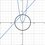# Basics Faster Than Skills

Factorize $(b-c)^{6}+(c-a)^{6}+(a-b)^{6}-9(a-b)^{2}(b-c)^{2}(c-a)^{2}-2(a-b)^{3}(a-c)^{3}-2(b-c)^{3}(b-a)^{3}-2(c-a)^{3}(c-b)^{3}$

• When you got the answer you must be surprised, feeling cheated.
• Think about the title carefully, in that there are two ways to solve it.Note by Jack Nicolson
3 years, 10 months ago

This discussion board is a place to discuss our Daily Challenges and the math and science related to those challenges. Explanations are more than just a solution — they should explain the steps and thinking strategies that you used to obtain the solution. Comments should further the discussion of math and science.

When posting on Brilliant:

• Use the emojis to react to an explanation, whether you're congratulating a job well done , or just really confused .
• Ask specific questions about the challenge or the steps in somebody's explanation. Well-posed questions can add a lot to the discussion, but posting "I don't understand!" doesn't help anyone.
• Try to contribute something new to the discussion, whether it is an extension, generalization or other idea related to the challenge.

MarkdownAppears as
*italics* or _italics_ italics
**bold** or __bold__ bold
- bulleted- list
• bulleted
• list
1. numbered2. list
1. numbered
2. list
Note: you must add a full line of space before and after lists for them to show up correctly
paragraph 1paragraph 2

paragraph 1

paragraph 2

[example link](https://brilliant.org)example link
> This is a quote
This is a quote
    # I indented these lines
# 4 spaces, and now they show
# up as a code block.

print "hello world"
# I indented these lines
# 4 spaces, and now they show
# up as a code block.

print "hello world"
MathAppears as
Remember to wrap math in $$ ... $$ or $ ... $ to ensure proper formatting.
2 \times 3 $2 \times 3$
2^{34} $2^{34}$
a_{i-1} $a_{i-1}$
\frac{2}{3} $\frac{2}{3}$
\sqrt{2} $\sqrt{2}$
\sum_{i=1}^3 $\sum_{i=1}^3$
\sin \theta $\sin \theta$
\boxed{123} $\boxed{123}$

Sort by:

Yes indeed...it looks quite symmetric doesn't it?

Let $a-b=x, b-c=y, c-a=z$

Then the expression becomes:

$x^6+y^6+z^6-9x^2y^2z^2+2x^3y^3+2y^3z^3+2z^3x^3$

$= (x^3+y^3+z^3)^2-(3xyz)^2$

$= (x^3+y^3+z^3-3xyz)(x^3+y^3+z^3+3xyz)$

Now we note that $x+y+z=0$ which means that $x^3+y^3+z^3=3xyz$ and our whole expression becomes 0

- 3 years, 10 months ago

That's why I said you'll be shocked :D, your method is faster than those I thought because I, in fact, want you guys to get it simplified step by step first, obviously you didn't jump into my trap :P

- 3 years, 10 months ago

0,same way as rohit.

- 3 years, 10 months ago

Aha, another brilliant person

- 3 years, 10 months ago

0

- 3 years, 10 months ago

Yeah, did it take you a long time? :D

- 3 years, 10 months ago

Certainly not....

- 3 years, 10 months ago

My advice to all regarding such long questions is to first try your luck with 0,and if it doesn't work,move to the next one

- 3 years, 10 months ago

Ha-ha, next time I'll try it, thanks for your nice method :D

- 3 years, 10 months ago